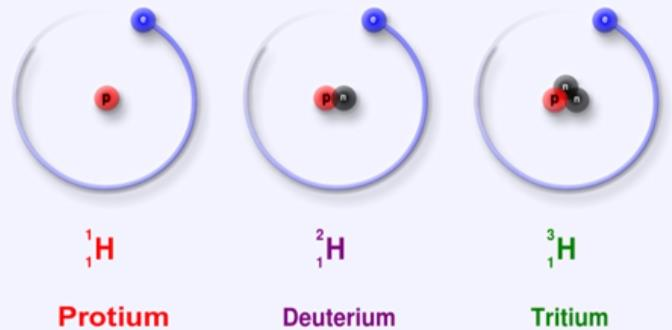Courses

# Atomic and Molecular Mass JEE Notes | EduRev

## JEE : Atomic and Molecular Mass JEE Notes | EduRev

The document Atomic and Molecular Mass JEE Notes | EduRev is a part of JEE category.
All you need of JEE at this link: JEE

An atom is such small a particle that it cannot be seen or isolated. Therefore, it is impossible to determine the actual mass of a single atom by weighing it. However, Avogadro’s hypothesis finally solved the problem. He took equal volumes of two different gases under similar conditions of temperature and pressure. He then weighed them.

Surprisingly, the ratio of their masses was equal to the ratio of their single molecules. Thus, though the actual masses of the atoms could not be determined, their relative masses could be determined. If the atomic mass of hydrogen is taken as 1, the relative atomic mass of oxygen is 16.

Initially, scientists obtained the atomic masses of all the elements by comparing with the mass of hydrogen taken as 1. However, the problem was that the atomic masses of most of the elements came out to be fractional in this method. Therefore, carbon is taken as the reference for the determination of atomic masses.

What's Actually Atomic Mass is ?

Atomic mass of an element is the number of times an atom of that element is heavier than an atom of carbon taken as 12. One atomic mass unit is equal to one-twelfth of the mass of an atom of carbon 12 isotope. The atomic mass of an element is the average relative mass of its atoms as compared to an atom of carbon 12 taken as 12.

Fractional abundance of an isotope is the fraction of the total number of atoms that is comprised of that particular isotope. Atomic mass of an element = (Fractional abundance of isotope 1 × mass of isotope 1) + (Fractional abundance of isotope 2 × mass of isotope 2).Gram Atomic Masses

The atomic masses of elements expressed in grams is their gram atomic masses. For eg: the atomic mass of oxygen is 16 amu.

∴ Gram atomic mass of oxygen = 16 g.

Molecular Mass

The molecular mass of a substance is the number of times the molecule of the substance is heavier than one-twelth the mass of an atom of carbon -12. Or, the molecular mass is equal to the sum of its atomic masses of all the atoms present in one molecule of a substance. For eg: Water (H2O).

Atomic mass of H= 1 unit

Atomic mass of O =16 units

The molecular mass of water = 2 × atomic mass of H+1 × atomic mass of O

= 2 × 1 + 16 × 1

= 18 units

Gram Molecular Mass

The molecular mass of a substance expressed in grams is the gram molecular mass. For eg: Molecular mass of oxygen = 32u

∴ Gram molecular mass of oxygen = 32 g.

Offer running on EduRev: Apply code STAYHOME200 to get INR 200 off on our premium plan EduRev Infinity!

,

,

,

,

,

,

,

,

,

,

,

,

,

,

,

,

,

,

,

,

,

;